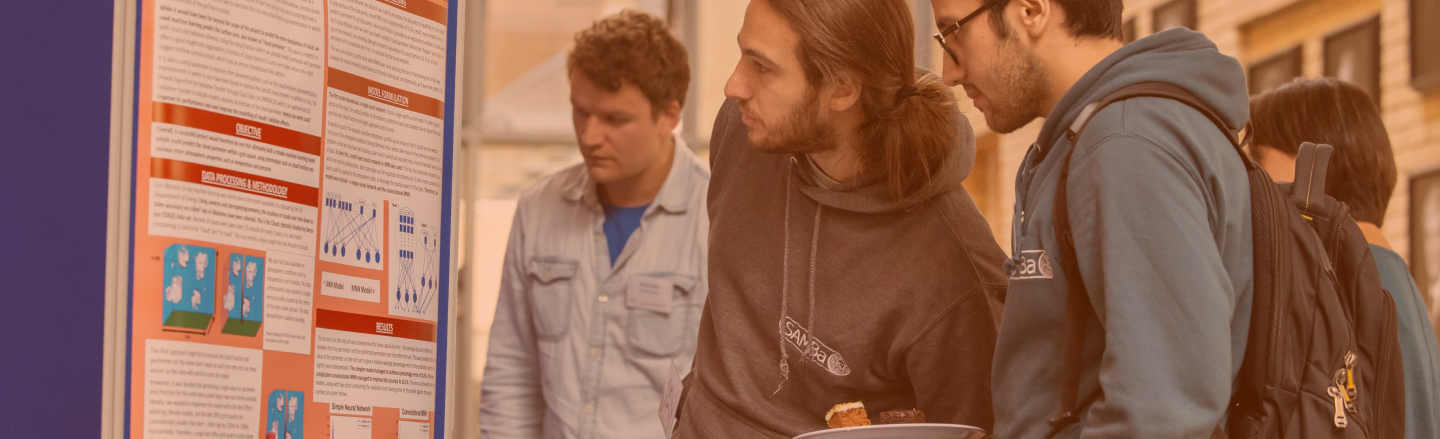#### Deep Learning as Optimal Control

The aim of this WP is to establish a mathematical framework for DL based on continuum models, such as neural ODEs. This investigation is based on the hypothesis that DNNs are discretisations of suitable continuum models, such as ODE systems, PDEs or integral equations. An illustration of this is shown above where dots represent data points nonlinearly transformed by a DRN network into a higher dimensional space (i.e. “lifting”), allowing for classification methods based on linear separability to be used effectively. This WP aims to rigorously investigate this connection, studying the properties of the continuum models, and exploring the various implications on the theoretical analysis of DL techniques, e.g., stability, expressivity and generalisability, using the toolkit of both smooth, and non-smooth, dynamical systems and optimal control theory.